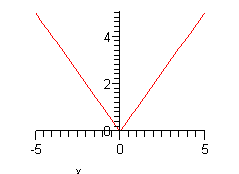Paul's Online Notes
Home / Algebra Trig Review / Algebra / Graphing and Common Graphs
Show Mobile Notice Show All Notes Hide All Notes
Mobile Notice
You appear to be on a device with a "narrow" screen width (i.e. you are probably on a mobile phone). Due to the nature of the mathematics on this site it is best views in landscape mode. If your device is not in landscape mode many of the equations will run off the side of your device (should be able to scroll to see them) and some of the menu items will be cut off due to the narrow screen width.

### Section 1.9 : Graphing and Common Graphs

Sketch the graph of each of the following. Show All Solutions Hide All Solutions

1. $$y = - \frac{2}{5}x + 3$$
Show Solution

This is a line in the slope intercept form

$y = mx + b$

In this case the line has a $$y$$ intercept of $$\left( {0,b} \right)$$ and a slope of $$m$$. Recall that slope can be thought of as

$m = \frac{\mbox{rise}}{\mbox{run}}$

If the slope is negative we tend to think of the rise as a fall.

The slope allows us to get a second point on the line. Once we have any point on the line and the slope we move right by run and up/down by rise depending on the sign. This will be a second point on the line.

In this case we know (0,3) is a point on the line and the slope is $$- \frac{2}{5}$$. So, starting at (0,3) we’ll move 5 to the right (i.e. $$0 \to 5$$) and down 2 (i.e. $$3 \to 1$$) to get (5,1) as a second point on the line. Once we’ve got two points on a line all we need to do is plot the two points and connect them with a line.

Here’s the sketch for this line.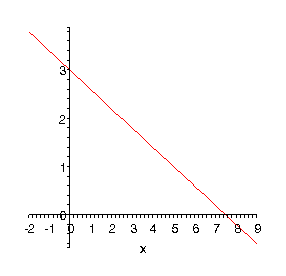2. $$y = {\left( {x + 3} \right)^2} - 1$$
Show Solution

This is a parabola in the form

$y = {\left( {x - h} \right)^2} + k$

Parabolas in this form will have the vertex at $$\left(h, k\right)$$. So, the vertex for our parabola is (-3,-1). We can also notice that this parabola will open up since the coefficient of the squared term is positive (and of course it would open down if it was negative).

In graphing parabolas, it is also convenient to have the $$x$$-intercepts, if they exist. In this case they will exist since the vertex is below the $$x$$-axis and the parabola opens up. To find them all we need to do is solve.

\begin{align*}{\left( {x + 3} \right)^2} - 1 & = 0\\ {\left( {x + 3} \right)^2} & = 1\\ x + 3 & = \pm 1\\ x &= - 4\,\,{\mbox{and }}\,x = - 2\end{align*}

With this information we can plot the main points and sketch in the parabola. Here is the sketch.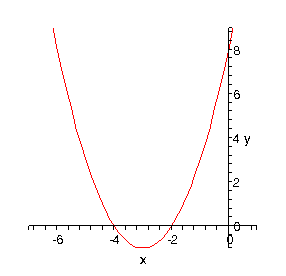3. $$y = - {x^2} + 2x + 3$$
Show Solution

This is also the graph of a parabola only it is in the more general form.

$y = a{x^2} + bx + c$

In this form, the $$x$$-coordinate of the vertex is $$x = - \frac{b}{{2a}}$$ and we get the $$y$$-coordinate by plugging this value back into the equation. So, for our parabola the coordinates of the vertex will be.

\begin{align*} x & = - \frac{2}{{2\left( { - 1} \right)}} = 1\\ y & = - {\left( 1 \right)^2} + 2\left( 1 \right) + 3 = 4\end{align*}

So, the vertex for this parabola is (1,4).

We can also determine which direction the parabola opens from the sign of $$a$$. If $$a$$ is positive the parabola opens up and if $$a$$ is negative the parabola opens down. In our case the parabola opens down.

This also means that we’ll have $$x$$-intercepts on this graph. So, we’ll solve the following.

\begin{align*} - {x^2} + 2x + 3 & = 0\\ {x^2} - 2x - 3 & = 0\\ \left( {x - 3} \right)\left( {x + 1} \right) & = 0\end{align*}

So, we will have $$x$$-intercepts at $$x = - 1$$ and $$x = 3$$. Notice that to make my life easier in the solution process I multiplied everything by -1 to get the coefficient of the $${x^2}$$ positive. This made the factoring easier.

Here’s a sketch of this parabola.We could also use completing the square to convert this into the form used in the previous problem. To do this I’ll first factor out a -1 since it’s always easier to complete the square if the coefficient of the $${x^2}$$ is a positive 1.

\begin{align*}y & = - {x^2} + 2x + 3\\ & = - \left( {{x^2} - 2x - 3} \right)\end{align*}

Now take half the coefficient of the $$x$$ and square that. Then add and subtract this to the quantity inside the parenthesis. This will make the first three terms a perfect square which can be factored.

\begin{align*}y & = - \left( {{x^2} - 2x - 3} \right)\\ & = - \left( {{x^2} - 2x + 1 - 1 - 3} \right)\\ & = - \left( {{x^2} - 2x + 1 - 4} \right)\\ & = - \left( {{{\left( {x - 1} \right)}^2} - 4} \right)\end{align*}

The final step is to multiply the minus sign back through.

$y = - {\left( {x - 1} \right)^2} + 4$
4. $$f\left( x \right) = - {x^2} + 2x + 3$$
Show Solution

The only difference between this problem and the previous problem is that we’ve got an $$f\left( x \right)$$ on the left instead of a $$y$$. However, remember that $$f\left( x \right)$$ is just a fancy way of writing the $$y$$ and so graphing $$f\left( x \right) = - {x^2} + 2x + 3$$ is identical to graphing $$y = - {x^2} + 2x + 3$$.

So, see the previous problem for the work. For completeness sake here is the graph.5. $$x = {y^2} - 6y + 5$$
Show Solution

Most people come out of an Algebra class capable of dealing with functions in the form $$y = f(x)$$. However, many functions that you will have to deal with in a Calculus class are in the form $$x = f(y)$$ and can only be easily worked with in that form. So, you need to get used to working with functions in this form.

The nice thing about these kinds of function is that if you can deal with functions in the form $$y = f(x)$$ then you can deal with functions in the form $$x = f(y)$$.

So,

$y = {x^2} - 6x + 5$

is a parabola that opens up and has a vertex of (3,-4).

Well our function is in the form

$x = a{y^2} + by + c$

and this is a parabola that opens left or right depending on the sign of $$a$$ (right if $$a$$ is positive and left if $$a$$ is negative). The $$y$$-coordinate of the vertex is given by $$y = - \frac{b}{{2a}}$$ and we find the $$x$$-coordinate by plugging this into the equation.

Our function is a parabola that opens to the right ($$a$$ is positive) and has a vertex at (-4,3). To graph this, we’ll need $$y$$-intercepts. We find these just like we found ­$$x$$-intercepts in the previous couple of problems.

\begin{align*}{y^2} - 6y + 5 & = 0\\ \left( {y - 5} \right)\left( {y - 1} \right) & = 0\end{align*}

The parabola will have ­$$y$$-intercepts at $$y = 1$$ and $$y = 5$$. Here’s a sketch of the graph.6. $${x^2} + {\left( {y + 5} \right)^2} = 4$$
Show Solution

This is a circle in it s standard form

${\left( {x - h} \right)^2} + {\left( {y - k} \right)^2} = {r^2}$

When circles are in this form we can easily identify the center, $$\left( {h,k} \right)$$, and radius, $$r$$. Once we have these we can graph the circle simply by starting at the center and moving right, left, up and down by $$r$$ to get the rightmost, leftmost, top most and bottom most points respectively.

Our circle has a center at (0, -5) and a radius of 2. Here’s a sketch of this circle.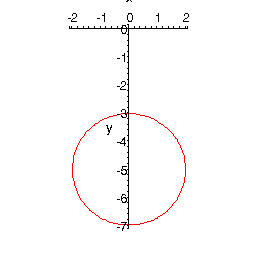7. $${x^2} + 2x + {y^2} - 8y + 8 = 0$$
Show Solution

This is also a circle, but it isn’t in standard form. To graph this, we’ll need to do it put it into standard form. That’s easy enough to do however. All we need to do is complete the square on the $$x$$’s and on the $$y$$’s.

\begin{align*}{x^2} + 2x + {y^2} - 8y + 8 & = 0\\ {x^2} + 2x + 1 - 1 + {y^2} - 8y + 16 - 16 + 8 & = 0\\ {\left( {x + 1} \right)^2} + {\left( {y - 4} \right)^2} & = 9\end{align*}

So, it looks like the center is (-1, 4) and the radius is 3. Here’s a sketch of the circle.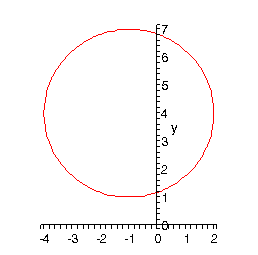8. $$\displaystyle \frac{{{{\left( {x - 2} \right)}^2}}}{9} + 4{\left( {y + 2} \right)^2} = 1$$
Show Solution

This is an ellipse. The standard form of the ellipse is

$\frac{{{{\left( {x - h} \right)}^2}}}{{{a^2}}} + \frac{{{{\left( {y - k} \right)}^2}}}{{{b^2}}} = 1$

This is an ellipse with center $$\left(h, k\right)$$ and the right most and left most points are a distance of $$a$$ away from the center and the top most and bottom most points are a distance of $$b$$ away from the center.

The ellipse for this problem has center (2, -2) and has $$a = 3$$ and $$b = \frac{1}{2}$$. Here’s a sketch of the ellipse.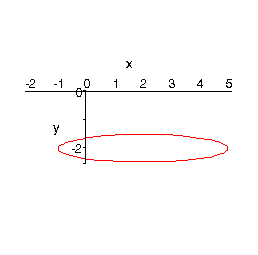9. $$\displaystyle \frac{{{{\left( {x + 1} \right)}^2}}}{9} - \frac{{{{\left( {y - 2} \right)}^2}}}{4} = 1$$
Show Solution

This is a hyperbola. There are two standard forms for a hyperbola.

 Form $$\displaystyle \frac{{{{\left( {x - h} \right)}^2}}}{{{a^2}}} - \frac{{{{\left( {y - k} \right)}^2}}}{{{b^2}}} = 1$$ $$\displaystyle \frac{{{{\left( {y - k} \right)}^2}}}{{{b^2}}} - \frac{{{{\left( {x - h} \right)}^2}}}{{{a^2}}} = 1$$ Center $$\left(h, k\right)$$ $$\left(h, k\right)$$ Opens Opens right and left Opens up and down Vertices $$a$$ units right and left of center $$b$$ units up and down from center Slope of Asymptotes $$\displaystyle \pm \frac{b}{a}$$ $$\displaystyle \pm \frac{b}{a}$$

So, what does all this mean? First, notice that one of the terms is positive and the other is negative. This will determine which direction the two parts of the hyperbola open. If the $$x$$ term is positive the hyperbola opens left and right. Likewise, if the $$y$$ term is positive the hyperbola opens up and down.

Both have the same “center”. Hyperbolas don’t really have a center in the sense that circles and ellipses have centers. The center is the starting point in graphing a hyperbola. It tells up how to get to the vertices and how to get the asymptotes set up.

The asymptotes of a hyperbola are two lines that intersect at the center and have the slopes listed above. As you move farther out from the center the graph will get closer and closer to they asymptotes.

For the equation listed here the hyperbola will open left and right. Its center is (-1, 2). The two vertices are (-4, 2) and (2, 2). The asymptotes will have slopes $$\pm \frac{2}{3}$$.

Here is a sketch of this hyperbola.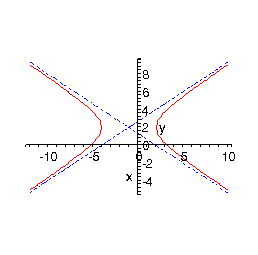10. $$y = \sqrt x$$
Show Solution

There isn’t much to this problem. This is just the square root and it’s a graph that you need to be able to sketch on occasion so here it is. Just remember that you can’t plug any negative $$x$$’s into the square root. Here is the graph.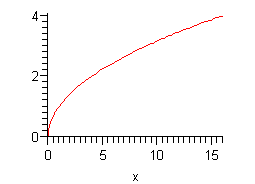11. $$y = {x^3}$$
Show Solution

Another simple graph that doesn’t really need any discussion, it is here simply to make sure you can sketch it.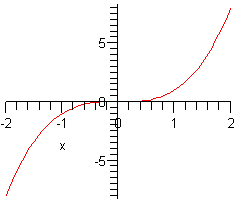12. $$y = \left| x \right|$$
Show Solution

This last sketch is not that difficult if you remember how to evaluate Absolute Value functions. Here is the sketch for this one.